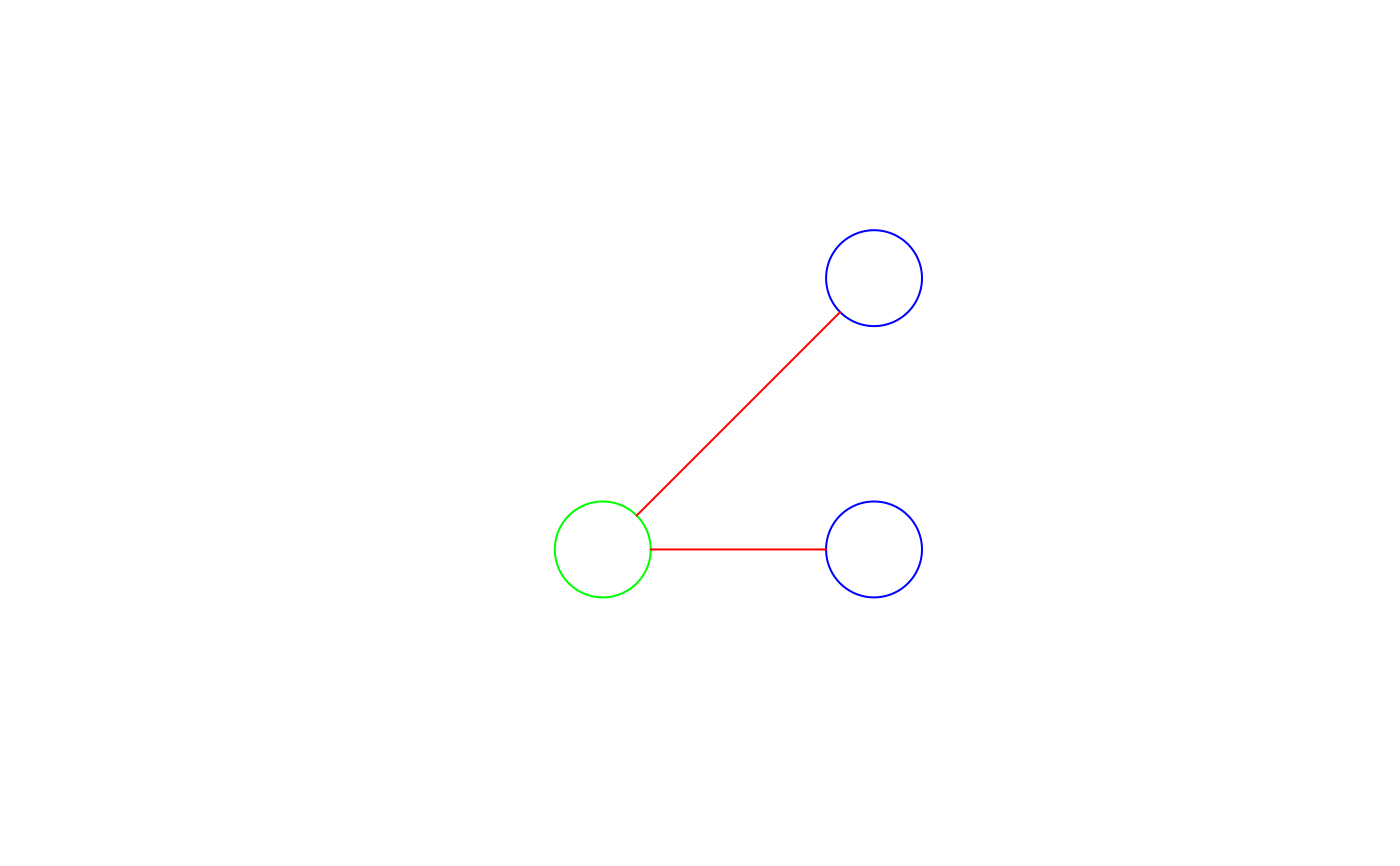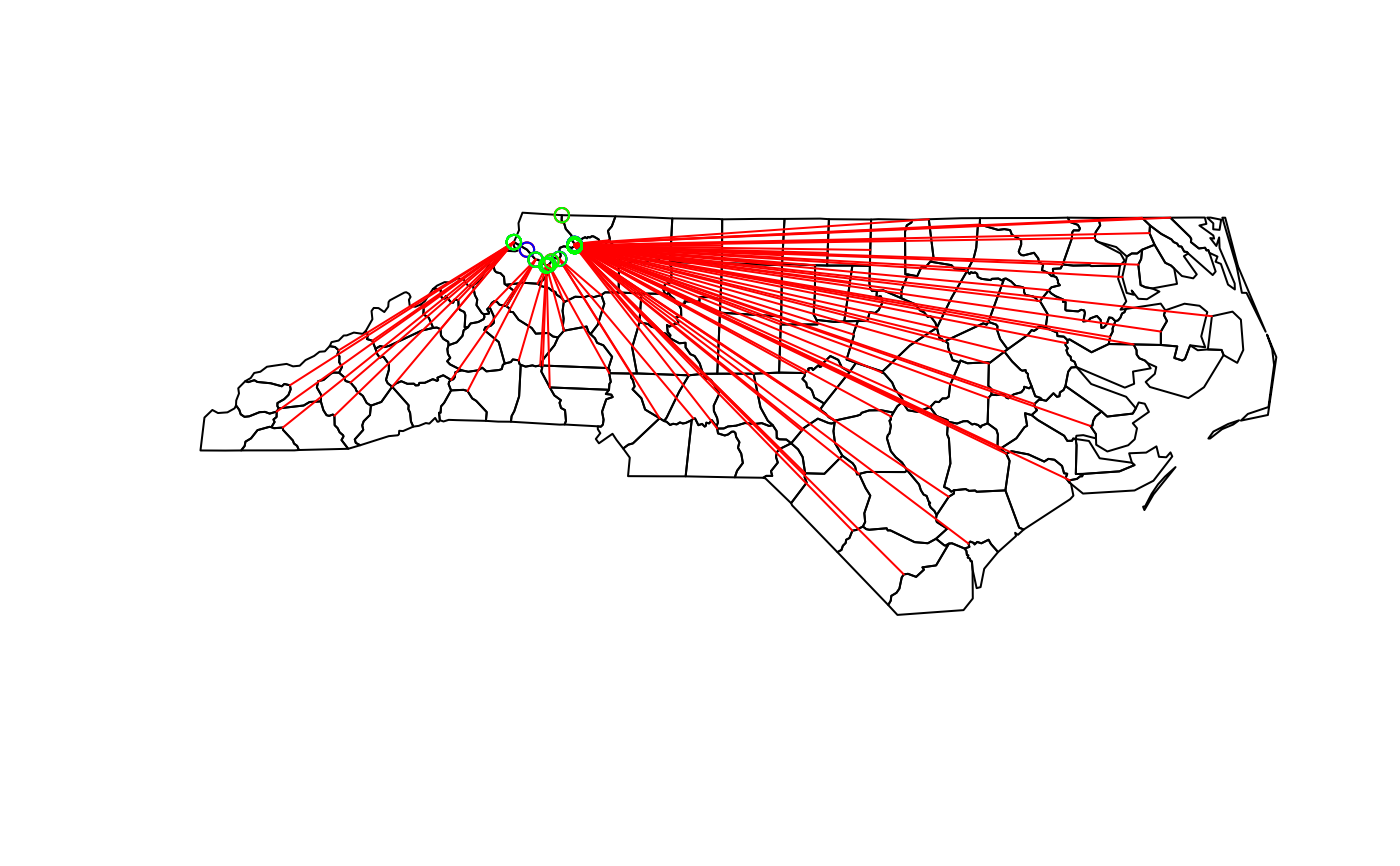get nearest points between pairs of geometries

st_nearest_points(x, y, ...)

# S3 method for sfc
st_nearest_points(x, y, ..., pairwise = FALSE)

# S3 method for sfg
st_nearest_points(x, y, ...)

# S3 method for sf
st_nearest_points(x, y, ...)

## Arguments

x object of class sfg, sfc or sf object of class sfg, sfc or sf ignored logical; if FALSE (default) return nearest points between all pairs, if TRUE, return nearest points between subsequent pairs.

## Value

an sfc object with all two-point LINESTRING geometries of point pairs from the first to the second geometry, of length x * y, with y cycling fastest. See examples for ideas how to convert these to POINT geometries.

st_nearest_feature for finding the nearest feature

## Examples

r = sqrt(2)/10
pt1 = st_point(c(.1,.1))
pt2 = st_point(c(.9,.9))
pt3 = st_point(c(.9,.1))
b1 = st_buffer(pt1, r)
b2 = st_buffer(pt2, r)
b3 = st_buffer(pt3, r)
(ls0 = st_nearest_points(b1, b2)) # sfg#> Geometry set for 1 feature
#> geometry type:  LINESTRING
#> dimension:      XY
#> bbox:           xmin: 0.2 ymin: 0.2 xmax: 0.8 ymax: 0.8
#> CRS:            NA#> LINESTRING (0.2 0.2, 0.8 0.8)(ls = st_nearest_points(st_sfc(b1), st_sfc(b2, b3))) # sfc#> Geometry set for 2 features
#> geometry type:  LINESTRING
#> dimension:      XY
#> bbox:           xmin: 0.2 ymin: 0.1 xmax: 0.8 ymax: 0.8
#> CRS:            NA#> LINESTRING (0.2 0.2, 0.8 0.8)#> LINESTRING (0.2414214 0.1, 0.7585786 0.1)plot(b1, xlim = c(-.2,1.2), ylim = c(-.2,1.2), col = NA, border = 'green')plot(st_sfc(b2, b3), add = TRUE, col = NA, border = 'blue')plot(ls, add = TRUE, col = 'red')plot(pts[seq(2, 200, 2)], add = TRUE, col = 'green')